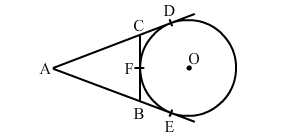# In the given figure, AD and AE are the tangents to a circle with centre O and BC touches the circle at F.`
Question:

In the given figure, AD and AE are the tangents to a circle with centre O and BC touches the circle at F. If AE = 5 cm, then perimeter of ∆ABC is(a) 15 cm
(b) 10 cm
(c) 22.5 cm
(d) 20 cm

Solution:

(b) 10 cm
Since the tangents from an external point are equal, we have:

$A D=A E, C D=C F, B E=B F$

Perimeter of $\Delta A B C=A C+A B+C B$

$=(A D-C D)+(C F+B F)+(A E-B E)$

$=(A D-C F)+(C F+B F)+(A E-B F)$

$=A D+A E$

$=2 A E$

$=2 \times 5$

$=10 \mathrm{~cm}$